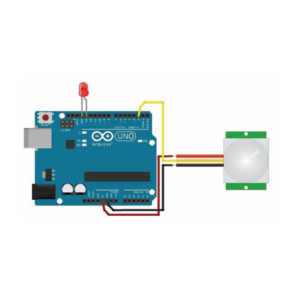# PIR Motion Sensor Arduino Project

Author: Aus Electronics Direct   Date Posted:9 April 2019# PIR Motion Sensor Arduino Project

This tutorial demonstrates how to detect motion from PIR Sensor using Arduino Uno Development Board. When the PIR detects, it will trigger the LED to light up showing as the output.

Electric Parts RequiredCode:

PIR Motion Sensor with Arudino Demo Code

*/

int led = 13;                // Define the LED as Pin 13

int sensor = 4;              // Define the Sensor Connected to Pin 4

int state = LOW;             // Motion Detection

int val = 0;                 // Store the value of sensor

void setup() {

pinMode(led, OUTPUT);      // initialize the LED as the output

pinMode(sensor, INPUT);    // initialize the sensor as the input

Serial.begin(9600);        // Define the serial communication

}

void loop(){

if (val == HIGH) {           // if sensor is high

digitalWrite(led, HIGH);   // switch on the LED

delay(100);                // 100 milliseconds delay

if (state == LOW) {

Serial.println("Motion was detected");

state = HIGH;       // Update the variable state in to HIGH

}

}

else {

digitalWrite(led, LOW); // Turning off the LED

delay(200);             // 200 milliseconds delay

if (state == HIGH){

Serial.println("Motion stopped!");

state = LOW;       // update the variable state into LOW

}

}

}